JMSLTM Numerical Library 7.2.0
com.imsl.stat

## Class ARMAOutlierIdentification

• All Implemented Interfaces:
Serializable, Cloneable

```public class ARMAOutlierIdentification
extends Object
implements Serializable, Cloneable```
Detects and determines outliers and simultaneously estimates the model parameters in a time series whose underlying outlier free series follows a general seasonal or nonseasonal ARMA model. This class also allows computation of forecasts.

Consider a univariate time series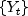that can be described by the following multiplicative seasonal ARIMA model of order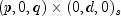: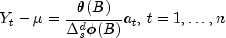Here,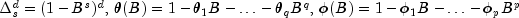. B is the lag operator,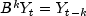,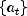is a white noise process, and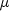denotes the mean of the series.

Outlier detection and parameter estimation

In general,is not directly observable due to the influence of outliers. Chen and Liu (1993) distinguish between four types of outliers: innovational outliers (IO), additive outliers (AO), temporary changes (TC) and level shifts (LS). If an outlier occurs as the last observation of the series, then Chen and Liu's algorithm is unable to determine the outlier's classification. In class `ARMAOutlierIdentification`, such an outlier is called a UI (unable to identify) and is treated as an innovational outlier.

In order to take the effects of multiple outliers occurring at time points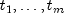into account, Chen and Liu consider the following model: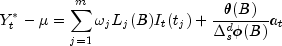Here,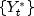is the observed outlier contaminated series, and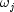and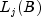denote the magnitude and dynamic pattern of outlier j, respectively.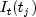is an indicator function that determines the temporal course of the outlier effect,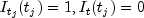otherwise. Note thatoperates on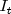via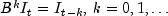.

The last formula shows that the outlier free seriescan be obtained from the original seriesby removing all occuring outlier effects: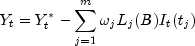The different types of outliers are charaterized by different values for:

1.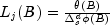for an innovational outlier,
2.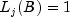for an additive outlier,
3.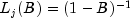for a level shift outlier and
4.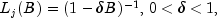for a temporary change outlier.

Class `ARMAOutlierIdentification` is an implementation of Chen and Liu's algorithm. It determines the coefficients in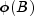and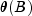and the outlier effects in the model for the observed series jointly in three stages. The magnitude of the outlier effects is determined by least squares estimates. Outlier detection itself is realized by examination of the maximum value of the standardized statistics of the outlier effects. For a detailed description, see Chen and Liu's original paper (1993).

Intermediate and final estimates for the coefficients inandare computed by the `compute` methods from JMSL classes `ARMA` and `ARMAMaxLikelihood`. If the roots oforlie on or within the unit circle, then the algorithm stops with an appropriate exception. In this case, different values for p and q should be tried.

Forecasting

From the relation between original and outlier free series,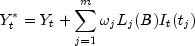it follows that the Box-Jenkins forecast at origin t for lead time l,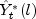, can be computed as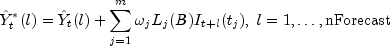Therefore, computation of the forecasts foris done in two steps:
1. Computation of the forecasts for the outlier free series.
2. Computation of the forecasts for the original seriesby adding the multiple outlier effects to the forecasts for.

Step 1: Computation of the forecasts for the outlier free seriesSince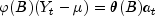where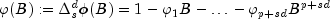the Box-Jenkins forecast at origin t for lead time l,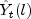, can be computed recursively as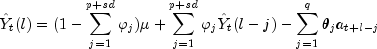Here,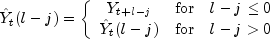and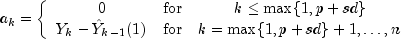Step 2: Computation of the forecasts for the original series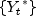by adding the multiple outlier effects to the forecasts forThe formulas forfor the different types of outliers are as follows:

Innovational outlier (IO)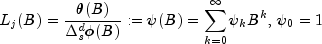Level shifts (LS)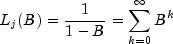Temporary changes (TC)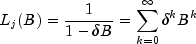Assuming the outlier occurs at time point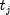, the outlier impact is therefore:

Innovational outliers (IO)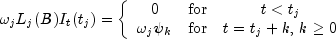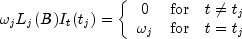Level shifts (LS)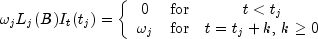Temporary changes (TC)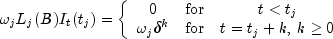From these formulas, the forecastscan be computed easily. The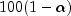percent probability limits for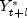and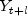are given by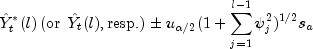where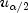is the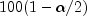percentile of the standard normal distribution,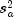is an estimate of the variance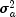of the random shocks, and the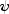weights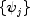are the coefficients in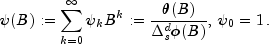For a detailed explanation of these concepts, see chapter 5:"Forecasting" in Box, Jenkins and Reinsel (1994).
Example 1, Example 2, Example 3, Serialized Form
• ### Field Summary

Fields
Modifier and Type Field and Description
`static int` `ADDITIVE`
Indicates detection of an additive outlier.
`static int` `INNOVATIONAL`
Indicates detection of an innovational outlier.
`static int` `LEVEL_SHIFT`
Indicates detection of a level shift outlier.
`static int` `TEMPORARY_CHANGE`
Indicates detection of a temporary change outlier.
`static int` `UNABLE_TO_IDENTIFY`
Indicates detection of an outlier that cannnot be categorized.
• ### Constructor Summary

Constructors
Constructor and Description
`ARMAOutlierIdentification(double[] z)`
Constructor for `ARMAOutlierIdentification`.
• ### Method Summary

Methods
Modifier and Type Method and Description
`void` `compute(int[] model)`
Detects and determines outliers and simultaneously estimates the model parameters for the given time series.
`void` `computeForecasts(int nForecast)`
Computes forecasts, associated probability limits andweights for an outlier contaminated time series whose underlying outlier free series obeys a general seasonal or non-seasonal ARMA model.
`double` `getAIC()`
Returns Akaike's information criterion (AIC).
`double` `getAICC()`
Returns Akaike's Corrected Information Criterion (AICC).
`double[]` `getAR()`
Returns the final autoregressive parameter estimates.
`double` `getBIC()`
Returns the Bayesian Information Criterion (BIC).
`double` `getConstant()`
Returns the constant parameter estimate.
`double[]` `getDeviations()`
Returns the deviations used for calculating the forecast confidence limits.
`double[]` `getForecast()`
Returns forecasts for the original outlier contaminated series.
`double[]` `getMA()`
Returns the final moving average parameter estimates.
`int` `getNumberOfOutliers()`
Returns the number of outliers detected.
`double[]` `getOmegaWeights()`
Returns theweights for the detected outliers.
`double[]` `getOutlierFreeForecast()`
Returns forecasts for the outlier free series.
`double[]` `getOutlierFreeSeries()`
Returns the outlier free series.
`int[][]` `getOutlierStatistics()`
Returns the outlier statistics.
`double[]` `getPsiWeights()`
Returns theweights of the infinite order moving average form of the model.
`double[]` `getResidual()`
Returns the residuals.
`double` `getResidualStandardError()`
Returns the residual standard error of the outlier free series.
`double[]` `getTauStatistics()`
Returns the t value for each detected outlier.
`void` `setAccuracyTolerance(double epsilon)`
Sets the tolerance value controlling the accuracy of the parameter estimates.
`void` `setConfidence(double confidence)`
Sets the confidence level for calculating confidence limit deviations returned from `getDeviations`.
`void` `setCriticalValue(double critical)`
Sets the critical value used as a threshold during outlier detection.
`void` `setDelta(double delta)`
Sets the dampening effect parameter.
`void` `setRelativeError(double relativeError)`
Sets the stopping criterion for use in the nonlinear equation solver.
• ### Methods inherited from class java.lang.Object

`clone, equals, finalize, getClass, hashCode, notify, notifyAll, toString, wait, wait, wait`
• ### Field Detail

`public static final int ADDITIVE`
Indicates detection of an additive outlier.
Constant Field Values
• #### INNOVATIONAL

`public static final int INNOVATIONAL`
Indicates detection of an innovational outlier.
Constant Field Values
• #### LEVEL_SHIFT

`public static final int LEVEL_SHIFT`
Indicates detection of a level shift outlier.
Constant Field Values
• #### TEMPORARY_CHANGE

`public static final int TEMPORARY_CHANGE`
Indicates detection of a temporary change outlier.
Constant Field Values
• #### UNABLE_TO_IDENTIFY

`public static final int UNABLE_TO_IDENTIFY`
Indicates detection of an outlier that cannnot be categorized.
Constant Field Values
• ### Constructor Detail

• #### ARMAOutlierIdentification

`public ARMAOutlierIdentification(double[] z)`
Constructor for `ARMAOutlierIdentification`.
Parameters:
`z` - a `double` array containing the observations.
• ### Method Detail

• #### compute

```public final void compute(int[] model)
throws ARMAMaxLikelihood.NonInvertibleException,
ARMAMaxLikelihood.NonStationaryException,
ARMAMaxLikelihood.InitialMAException,
ZeroPolynomial.DidNotConvergeException,
ARMA.MatrixSingularException,
ARMA.TooManyCallsException,
ARMA.IncreaseErrRelException,
ARMA.NewInitialGuessException,
ARMA.IllConditionedException,
ARMA.TooManyITNException,
ARMA.TooManyFcnEvalException,
ARMA.TooManyJacobianEvalException,
SingularMatrixException,
Cholesky.NotSPDException```
Detects and determines outliers and simultaneously estimates the model parameters for the given time series.
Parameters:
`model` - an `int` array of length 4 containing the numbers p, q, s, d of the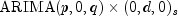model the outlier free series is following. It is required that p, q and d are non-negative and s is positive and consistent with `z.length`.
Throws:
`ARMAMaxLikelihood.NonStationaryException` - is thrown if the intermediate or final maximum likelihood estimates for the time series are nonstationary.
`ARMAMaxLikelihood.NonInvertibleException` - is thrown if the intermediate or final maximum likelihood estimates for the time series are noninvertible.
`ARMAMaxLikelihood.InitialMAException` - is thrown if the initial values provided for the moving average terms are noninvertible. In this case, ```ARMAMaxLikelihood ``` terminates and does not compute the time series estimates.
`ZeroPolynomial.DidNotConvergeException` - is thrown if the algorithm computing the roots of the AR- or MA- polynomial does not converge.
`ARMA.MatrixSingularException` - is thrown if the input matrix is singular.
`ARMA.TooManyCallsException` - is thrown if the number of calls to the function has exceeded the maximum number of iterations times the number of moving average (MA) parameters + 1.
`ARMA.IncreaseErrRelException` - is thrown if the bound for the relative error is too small.
`ARMA.NewInitialGuessException` - is thrown if the iteration has not made good progress.
`ARMA.IllConditionedException` - is thrown if the problem is ill-conditioned.
`ARMA.TooManyITNException` - is thrown if the maximum number of iterations is exceeded.
`ARMA.TooManyFcnEvalException` - is thrown if the maximum number of function evaluations is exceeded.
`ARMA.TooManyJacobianEvalException` - is thrown if the maximum number of Jacobian evaluations is exceeded.
`SingularMatrixException` - is thrown if during the computation of a small perturbation of the matrix product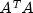, it is found that A, the matrix used in the determination of theweights, is singular.
`Cholesky.NotSPDException` - is thrown if during the computation of a small perturbation of the matrix product, it is found that A, the matrix used in the determination of theweights, is not positive definite.
• #### computeForecasts

`public final void computeForecasts(int nForecast)`
Computes forecasts, associated probability limits andweights for an outlier contaminated time series whose underlying outlier free series obeys a general seasonal or non-seasonal ARMA model.
Parameters:
`nForecast` - an `int` scalar containing the maximum lead time for forecasts. `nForecast` must be greater than 0. Forecast origin is the time point of the last observed value in the time series,. Forecasts are computed for lead times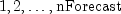, i.e. time points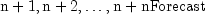. Note that the `compute` method must be invoked first before invoking this method. Otherwise, the method throws an `IllegalStateException` exception.
• #### getAIC

`public double getAIC()`
Returns Akaike's information criterion (AIC).
Returns:
a `double` scalar containing Akaike's information criterion (AIC) for the outlier free series. The `compute` method must be called before invoking this method. Otherwise, an `IllegalStateException` exception is thrown.
• #### getAICC

`public double getAICC()`
Returns Akaike's Corrected Information Criterion (AICC).
Returns:
a `double` scalar containing Akaike's Corrected Information Criterion (AICC) for the outlier free series. The `compute` method must be called before invoking this method. Otherwise, an `IllegalStateException` exception is thrown.
• #### getAR

`public double[] getAR()`
Returns the final autoregressive parameter estimates.
Returns:
a `double` array of length p = ```model ``` containing the final autoregressive parameter estimates. Note that the `compute` method must be invoked first before invoking this method. Otherwise, the method throws an `IllegalStateException` exception.
• #### getBIC

`public double getBIC()`
Returns the Bayesian Information Criterion (BIC).
Returns:
a `double` scalar containing the Bayesian Information Criterion (BIC) for the outlier free series. The `compute` method must be called before invoking this method. Otherwise, an `IllegalStateException` exception is thrown.
• #### getConstant

`public double getConstant()`
Returns the constant parameter estimate.
Returns:
a `double` scalar containing the constant parameter estimate. The `compute` method must be invoked first before invoking this method. Otherwise, the method throws an `IllegalStateException` exception.
• #### getDeviations

`public double[] getDeviations()`
Returns the deviations used for calculating the forecast confidence limits.
Returns:
a `double` array of length `nForecast` containing the deviations from each forecast for calculating forecast confidence intervals. The confidence level is specified in `setConfidence`. Method `computeForecasts` has to be invoked before this method is called. Otherwise, an `IllegalStateException` exception is thrown.
• #### getForecast

`public double[] getForecast()`
Returns forecasts for the original outlier contaminated series.
Returns:
a `double` array of length `nForecast` containing the forecasts for the original series. Method `computeForecasts` has to be invoked before this method is called. Otherwise, an `IllegalStateException` exception is thrown.
• #### getMA

`public double[] getMA()`
Returns the final moving average parameter estimates.
Returns:
a `double` array of length q = ```model ``` containing the final moving average parameter estimates. Note that the `compute` method must be invoked first before invoking this method. Otherwise, the method throws an `IllegalStateException` exception.
• #### getNumberOfOutliers

`public int getNumberOfOutliers()`
Returns the number of outliers detected.
Returns:
an `int` scalar containing the number of outliers detected. The `compute` method must be invoked first before invoking this method. Otherwise, an `IllegalStateException` exception is thrown.
• #### getOmegaWeights

`public double[] getOmegaWeights()`
Returns theweights for the detected outliers.
Returns:
a `double` array containing the computedweights for the detected outliers. If the number of detected outliers equals zero, then an array of length zero is returned. The `compute` method must be invoked before using this method. Otherwise, an `IllegalStateException` exception is thrown.
• #### getOutlierFreeForecast

`public double[] getOutlierFreeForecast()`
Returns forecasts for the outlier free series.
Returns:
a `double` array of length `nForecast` containing the forecasts for the outlier free series. Method `computeForecasts` has to be invoked before this method is called. Otherwise, an `IllegalStateException` exception is thrown.
• #### getOutlierFreeSeries

`public double[] getOutlierFreeSeries()`
Returns the outlier free series.
Returns:
a `double` array containing the outlier free series. The `compute` method must be called before invoking this method. Otherwise, an `IllegalStateException` exception is thrown.
• #### getOutlierStatistics

`public int[][] getOutlierStatistics()`
Returns the outlier statistics.
Returns:
an `int` matrix of length `nOutliers` by 2, where `nOutliers` is the number of detected outliers, containing the outlier statistics. The first column contains the time at which the outlier was observed (time ranging from 1 to `z.length`, the number of observations in the time series) and the second column contains an identifier indicating the type of outlier observed. Outlier types fall into one of five categories:  Identifier Outlier type `INNOVATIONAL` Innovational Outliers (IO) `ADDITIVE` Additive Outliers (AO) `LEVEL_SHIFT` Level Shift Outliers (LS) `TEMPORARY_CHANGE` Temporary Change Outliers (TC) `UNABLE_TO_IDENTIFY` Unable to Identify (UI)

If the number of detected outliers equals zero, then an ``` int``` array of size 0 is returned.

The `compute` method must be invoked first before invoking this method. Otherwise, an ```IllegalStateException ``` exception is thrown.
• #### getPsiWeights

`public double[] getPsiWeights()`
Returns theweights of the infinite order moving average form of the model.
Returns:
a `double` array of length `nForecast` containing theweights of the infinite order moving average form of the model for the outlier free series. Method `computeForecasts` must be invoked before this method is called. Otherwise, an `IllegalStateException` exception is thrown.
• #### getResidual

`public double[] getResidual()`
Returns the residuals.
Returns:
a `double` array containing the residuals for the outlier free series at the final parameter estimation point. The `compute` method must be invoked first before invoking this method. Otherwise, the method throws an `IllegalStateException` exception.
• #### getResidualStandardError

`public double getResidualStandardError()`
Returns the residual standard error of the outlier free series.
Returns:
a `double` scalar containing the standard error of the outlier free series. Note that the `compute` method must be invoked first before invoking this method. Otherwise, an `IllegalStateException` exception is thrown.
• #### getTauStatistics

`public double[] getTauStatistics()`
Returns the t value for each detected outlier.
Returns:
a `double` array containing the t statistics for each detected outlier. If the number of detected outliers equals zero, then a vector of length 0 is returned. The `compute` method must be invoked before using this method. Otherwise, an `IllegalStateException` exception is thrown.
• #### setAccuracyTolerance

`public void setAccuracyTolerance(double epsilon)`
Sets the tolerance value controlling the accuracy of the parameter estimates.
Parameters:
`epsilon` - a `double` scalar, a positive tolerance value controlling the accuracy of parameter estimates during outlier detection. Default: `epsilon = 0.001`.
• #### setConfidence

`public void setConfidence(double confidence)`
Sets the confidence level for calculating confidence limit deviations returned from `getDeviations`.
Parameters:
`confidence` - a `double` scalar specifying the confidence level used in computing forecast confidence intervals. Typical choices for `confidence` are 0.90, 0.95, and 0.99. `confidence` must be greater than 0.0 and less than 1.0. Default: `confidence = 0.95`.
• #### setCriticalValue

`public void setCriticalValue(double critical)`
Sets the critical value used as a threshold during outlier detection.
Parameters:
`critical` - a `double` scalar, the critical value used as a threshold for the statistics used in the outlier detection. `critical` must be greater than zero. Default: `critical = 3.0`.
• #### setDelta

`public void setDelta(double delta)`
Sets the dampening effect parameter.
Parameters:
`delta` - a `double` scalar, the dampening effect parameter used in the detection of a Temporary Change Outlier (TC). `delta` must be greater than 0 and less than 1. Default: `delta = 0.7`.
• #### setRelativeError

`public void setRelativeError(double relativeError)`
Sets the stopping criterion for use in the nonlinear equation solver.
Parameters:
`relativeError` - a `double` positive scalar containing the stopping criterion for use in the nonlinear equation solver used in the least-squares algorithm.
Default: ```relativeError= 1.0e-10```.
JMSLTM Numerical Library 7.2.0